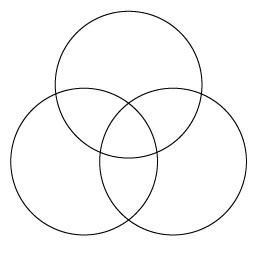# VENN DIAGRAM URGENT !!!

A Venn diagram is a common way for teachers and students to organize information. Have you ever seen a three-circle Venn diagram?a) Create a Venn diagram by writing equations for three circles. Your equations do not have to create a perfect replica of this image, but your circles must overlap in a similar way. It may help you to visualize your equations if you graph them! What are the equations to your circles?

b) What are coordinates of the centers of your circles? Assign each center a name.

c)What is the distance between each pair of centers? Show how you arrived at your answer.

d) If you choose two of your circle equations and solve them, how many solutions will you find?

e)Now consider all three circles together. How many solutions would you find if you were to solve them all as one system? Explain how you know your answer is correct.